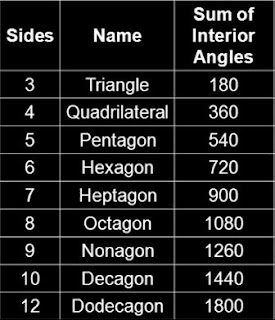## Cipher## Jun 23, 2017

### Spelling of numbers in English language

I made this small discovery about the spelling of numbers a few months ago but I forgot to make a post about it :) We are gonna look at the numbers from 0-9 and you'll see how they should be organized

First I wanna talk about the significance of number 9 for a second
-Any number reduced by it's reflection will always reduce to 9 ....478 - 874 = -396 
-Any number reduced by the sum of it's digits reduces to 9.... 478 - (4+7+8) = 459 
-9 multiplied by any number will always reduce to 9.... 478*9 = 4302 
Also sum of the angles of all regular polygons reduce to 9When we count to 9 we say 36 (9) letters
one two three four five six seven eight nine = 3+4+4 + 7+4+3 + 7+1+9+4+4 + 3+3+6+9 + 3+9+5+4 + 8+9+3 + 8+4+5+4+4 + 4+9+2+1+7 + 4+9+4+4 = 181 (Reverse Full Reduction)
thirty six letters = 7+19+18+9+7+2 + 8+18+3 + 15+22+7+7+22+9+8 = 181 (Reverse Ordinal)
181 is the 42nd prime number - Nine = 14+9+14+5 = 42 (English Ordinal)

If we take a look at the repeating Fibonacci pattern of 24 digits we find that numbers come in pairs, (1 and 8, 2 and 7, 3 and 6, 4 and 5) all of the pairs adding up to 9

So when we look at the spelling of numbers we just have to put the numbers that are paired next to each other to reveal the secret
-  every pair of numbers that add to 9 will have a total of 8 letters
-  8th triangular number is 36 = 3+6 = 9
Zero + Nine = 8 letters - (0+9 = 9)
One + Eight = 8 letters - (1+8 = 9)
Two + Seven = 8 letters - (2+7 = 9)
Three + Six = 8 letters - (3+6 = 9)
Four + Five = 8 letters - (4+5 = 9)

One Two Three Four Five Six Seven Eight Nine Ten = 6+5+5 + 2+5+6 + 2+8+9+5+5 + 6+6+3+9 + 6+9+4+5 + 10+9+6 + 10+5+4+5+5 + 5+9+7+8+2 + 5+9+5+5 + 2+5+5 = 227 (Single Reduction)
22/7 = 3.14 (Pi), the sum of the first 10 numbers is very fitting considering how we close the circle with 10
Twenty two divided by seven = 20+23+5+14+20+25 + 20+23+15 + 4+9+22+9+4+5+4 + 2+25 + 19+5+22+5+14 = 314 (English Ordinal)

Three hundred sixty = 20+8+18+5+5 + 8+21+14+4+18+5+4 + 19+9+24+20+25 = 227 (English Ordinal)